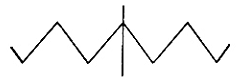# Consider a bundled conductor of an overhead line, consisting of three identical sub conductors placed at the corners of an equilateral triangle as shown in the figure. If we neglect the charges on the other phase conductors and ground, and assume that spacing between sub-conductors is much larger than their radius, the maximum electric field intensity is experienced at

1.  Point X

2.  Point Y

3.  Point Z

4.  Point W

4

Point Y

Explanation :
No Explanation available for this question

# The circuit shown in the figure is

1.  a voltage source with voltage (rV)//(R1//R2)

2.  a voltage source with voltage ((r//R2)/(R1))V

3.  a current source with current ((r//R2)/(R1 + R2)).V/r

4.  a current source with current (R2/(R1 + R2)).V/r

4

a current source with current (R2/(R1 + R2)).V/r

Explanation :
No Explanation available for this question

# The system shown in the figure is

1.  stable

2.  unstable

3.  conditionally stable

4.  stable for input u1, but unstable for input u2

4

stable for input u1, but unstable for input u2

Explanation :
No Explanation available for this question

# Let a signal a1 sin (ωlt + 1) be applied to a stable linear time - invariant system. Let the corresponding steady state output be represented as a2F (ω2t + ?2). Then which of the following statements is true?

1.  F is not necessarily a "sine" or "cosine" function but must be periodic with ωl = ω2

2.  F must be a "sine" or "cosine" function with a1 = a2

3.  F must be a "sine" function with ω1 = ω2 and 1 = ?2

4.  F must be a "sine" or "cosine" function with ω1 =ω2

4

F must be a "sine" or "cosine" function with ω1 =ω2

Explanation :
No Explanation available for this question

# The frequency spectrum of a signal is shown in the figure.If this signal is ideally sampled at intervals of 1 ms, then the frequency spectrum of the sampled signal will be

1.

2.

3.

4.

4Explanation :
No Explanation available for this question

# Divergence of the vector field V (x, y, z) = - (x cos xy + y) i + (y cos xy) j + (sin z2 + x2 + y2) k is

1.  2z cos z2

2.  sin xy + 2z cos z2

3.  x sin xy - cos z

4.   none of these

4

2z cos z2

Explanation :
No Explanation available for this question

# x = [x1 x2 ... xn]T is an n-tuple nonzero vector. The n × n matrix V = xxT

1.  has rank zero

2.  has rank l

3.  is orthogonal

4.  is orthogonal

4

is orthogonal

Explanation :
No Explanation available for this question

# A single - phase fully controlled thyristor bridge ac - dc converter is operating at a firing angle of 25° and an overlap angle 10° with constant dc output current of 20 A. The fundamental power factor (displacement factor) at input ac mains is

1.  0.78

2.  0.827

3.  0.866

4.  0.9

4

0.78

Explanation :
No Explanation available for this question

# A three - phase, fully controlled thyristor bridge converter is used as line commutated inverter to feed 50 kW power at 420 V dc to a three - phase, 415 V (line), 50 Hz ac mains. Consider dc link current to be constant. The rms current of the thyristor is

1.  119.05 A

2.  79.37 A

3.  68.73 A

4.  39.68 A

4

39.68 A

Explanation :
No Explanation available for this question

# In a transformer zero voltage regulation at full load is

1.  not possible

2.  possible at unity power factor load

4.  possible at lagging power factor load

4